plot one or more attributes of an sf object on a map Plot sf object

## Usage

# S3 method for sf
plot(
x,
y,
...,
main,
pal = NULL,
nbreaks = 10,
breaks = "pretty",
max.plot = if (is.null(n <- getOption("sf_max.plot"))) 9 else n,
key.pos = get_key_pos(x, ...),
key.length = 0.618,
key.width = lcm(1.8 * par("ps")/12),
reset = TRUE,
logz = FALSE,
extent = x,
xlim = st_bbox(extent)[c(1, 3)],
ylim = st_bbox(extent)[c(2, 4)]
)

get_key_pos(x, ...)

# S3 method for sfc_POINT
plot(
x,
y,
...,
pch = 1,
cex = 1,
col = 1,
bg = 0,
lwd = 1,
lty = 1,
type = "p",
)

# S3 method for sfc_MULTIPOINT
plot(
x,
y,
...,
pch = 1,
cex = 1,
col = 1,
bg = 0,
lwd = 1,
lty = 1,
type = "p",
)

# S3 method for sfc_LINESTRING
plot(x, y, ..., lty = 1, lwd = 1, col = 1, pch = 1, type = "l", add = FALSE)

# S3 method for sfc_CIRCULARSTRING
plot(x, y, ...)

# S3 method for sfc_MULTILINESTRING
plot(x, y, ..., lty = 1, lwd = 1, col = 1, pch = 1, type = "l", add = FALSE)

# S3 method for sfc_POLYGON
plot(
x,
y,
...,
lty = 1,
lwd = 1,
col = NA,
cex = 1,
pch = NA,
border = 1,
rule = "evenodd",
xpd = par("xpd")
)

# S3 method for sfc_MULTIPOLYGON
plot(
x,
y,
...,
lty = 1,
lwd = 1,
col = NA,
border = 1,
rule = "evenodd",
xpd = par("xpd")
)

# S3 method for sfc_GEOMETRYCOLLECTION
plot(
x,
y,
...,
pch = 1,
cex = 1,
bg = 0,
lty = 1,
lwd = 1,
col = 1,
border = 1,
)

# S3 method for sfc_GEOMETRY
plot(
x,
y,
...,
pch = 1,
cex = 1,
bg = 0,
lty = 1,
lwd = 1,
col = ifelse(st_dimension(x) == 2, NA, 1),
border = 1,
)

# S3 method for sfg
plot(x, ...)

plot_sf(
x,
xlim = NULL,
ylim = NULL,
asp = NA,
axes = FALSE,
bgc = par("bg"),
...,
xaxs,
yaxs,
lab,
setParUsrBB = FALSE,
bgMap = NULL,
expandBB = c(0, 0, 0, 0),
graticule = NA_crs_,
col_graticule = "grey",
border,
extent = x
)

sf.colors(n = 10, cutoff.tails = c(0.35, 0.2), alpha = 1, categorical = FALSE)

## Arguments

x

object of class sf

y

ignored

...

further specifications, see plot_sf and plot and details.

main

title for plot (NULL to remove)

pal

palette function, similar to rainbow, or palette values; if omitted, sf.colors is used

nbreaks

number of colors breaks (ignored for factor or character variables)

breaks

either a numeric vector with the actual breaks, or a name of a method accepted by the style argument of classIntervals

max.plot

integer; lower boundary to maximum number of attributes to plot; the default value (9) can be overriden by setting the global option sf_max.plot, e.g. options(sf_max.plot=2)

key.pos

numeric; side to plot a color key: 1 bottom, 2 left, 3 top, 4 right; set to NULL to omit key completely, 0 to only not plot the key, or -1 to select automatically. If multiple columns are plotted in a single function call by default no key is plotted and every submap is stretched individually; if a key is requested (and col is missing) all maps are colored according to a single key. Auto select depends on plot size, map aspect, and, if set, parameter asp. If it has lenght 2, the second value, ranging from 0 to 1, determines where the key is placed in the available space (default: 0.5, center).

key.length

amount of space reserved for the key along its axis, length of the scale bar

key.width

amount of space reserved for the key (incl. labels), thickness/width of the scale bar

reset

logical; if FALSE, keep the plot in a mode that allows adding further map elements; if TRUE restore original mode after plotting sf objects with attributes; see details.

logz

logical; if TRUE, use log10-scale for the attribute variable. In that case, breaks and at need to be given as log10-values; see examples.

extent

object with an st_bbox method to define plot extent; defaults to x

xlim

see plot.window

ylim

see plot.window

pch

plotting symbol

cex

symbol size

col

color for plotting features; if length(col) does not equal 1 or nrow(x), a warning is emitted that colors will be recycled. Specifying col suppresses plotting the legend key.

bg

symbol background color

lwd

line width

lty

line type

type

plot type: 'p' for points, 'l' for lines, 'b' for both

logical; add to current plot? Note that when using add=TRUE, you may have to set reset=FALSE in the first plot command.

border

color of polygon border(s); using NA hides them

rule

see polypath; for winding, exterior ring direction should be opposite that of the holes; with evenodd, plotting is robust against misspecified ring directions

xpd

see par; sets polygon clipping strategy; only implemented for POLYGON and MULTIPOLYGON

asp

see below, and see par

axes

logical; should axes be plotted? (default FALSE)

bgc

background color

xaxs

see par

yaxs

see par

lab

see par

setParUsrBB

default FALSE; set the par “usr” bounding box; see below

bgMap

object of class ggmap, or returned by function RgoogleMaps::GetMap

expandBB

numeric; fractional values to expand the bounding box with, in each direction (bottom, left, top, right)

graticule

logical, or object of class crs (e.g., st_crs(4326) for a WGS84 graticule), or object created by st_graticule; TRUE will give the WGS84 graticule or object returned by st_graticule

col_graticule

color to used for the graticule (if present)

n

integer; number of colors

cutoff.tails

numeric, in [0,0.5] start and end values

alpha

numeric, in [0,1], transparency

categorical

logical; do we want colors for a categorical variable? (see details)

## Details

plot.sf maximally plots max.plot maps with colors following from attribute columns, one map per attribute. It uses sf.colors for default colors. For more control over placement of individual maps, set parameter mfrow with par prior to plotting, and plot single maps one by one; note that this only works in combination with setting parameters key.pos=NULL (no legend) and reset=FALSE.

plot.sfc plots the geometry, additional parameters can be passed on to control color, lines or symbols.

When setting reset to FALSE, the original device parameters are lost, and the device must be reset using dev.off() in order to reset it.

parameter at can be set to specify where labels are placed along the key; see examples.

The features are plotted in the order as they apppear in the sf object. See examples for when a different plotting order is wanted.

plot_sf sets up the plotting area, axes, graticule, or webmap background; it is called by all plot methods before anything is drawn.

The argument setParUsrBB may be used to pass the logical value TRUE to functions within plot.Spatial. When set to TRUE, par(“usr”) will be overwritten with c(xlim, ylim), which defaults to the bounding box of the spatial object. This is only needed in the particular context of graphic output to a specified device with given width and height, to be matched to the spatial object, when using par(“xaxs”) and par(“yaxs”) in addition to par(mar=c(0,0,0,0)).

The default aspect for map plots is 1; if however data are not projected (coordinates are long/lat), the aspect is by default set to 1/cos(My * pi/180) with My the y coordinate of the middle of the map (the mean of ylim, which defaults to the y range of bounding box). This implies an Equirectangular projection.

non-categorical colors from sf.colors were taken from bpy.colors, with modified cutoff.tails defaults If categorical is TRUE, default colors are from https://colorbrewer2.org/ (if n < 9, Set2, else Set3).

## Examples

nc = st_read(system.file("gpkg/nc.gpkg", package="sf"), quiet = TRUE)
# plot single attribute, auto-legend:
plot(nc["SID74"])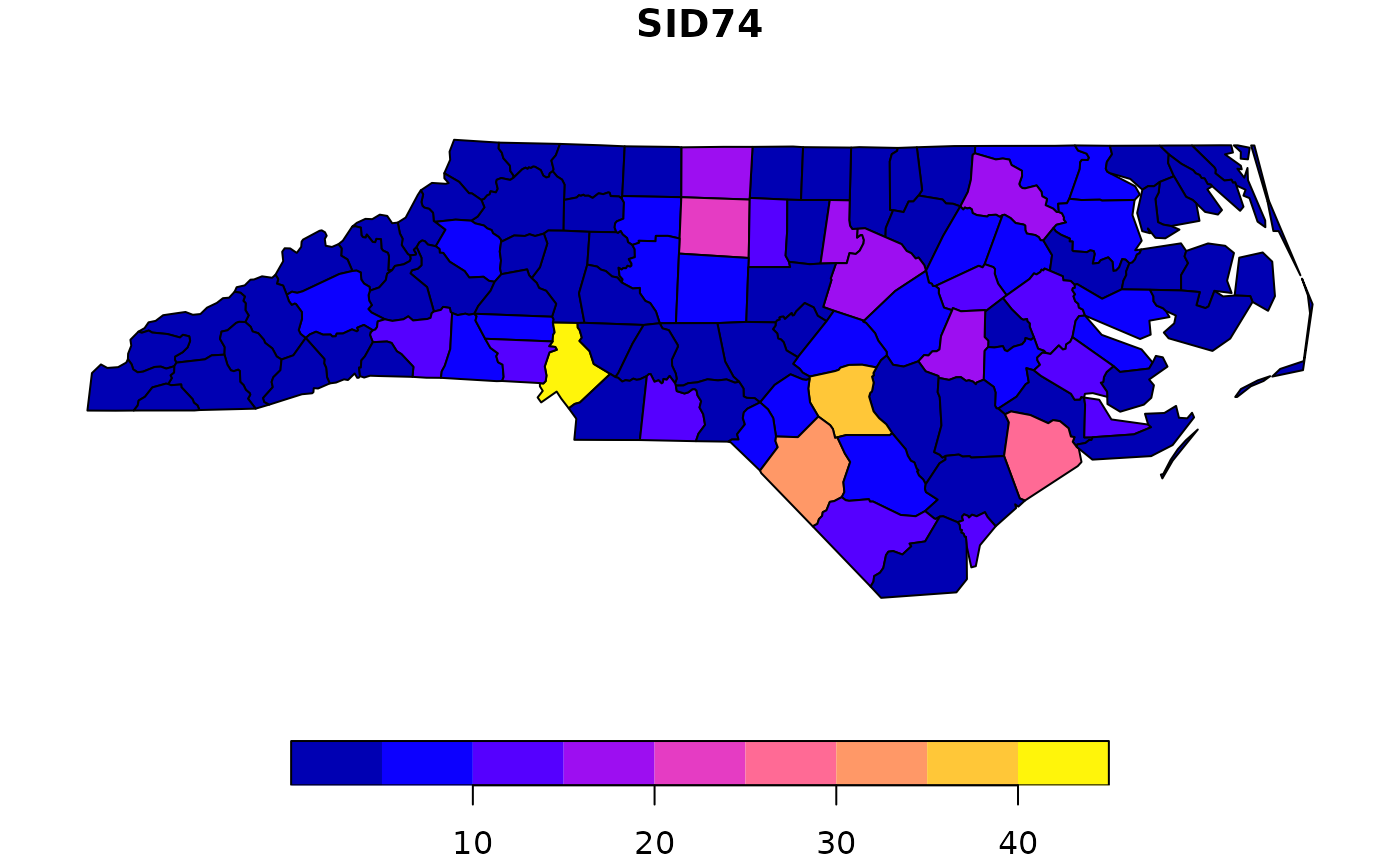# plot multiple:
plot(nc[c("SID74", "SID79")]) # better use ggplot2::geom_sf to facet and get a single legend!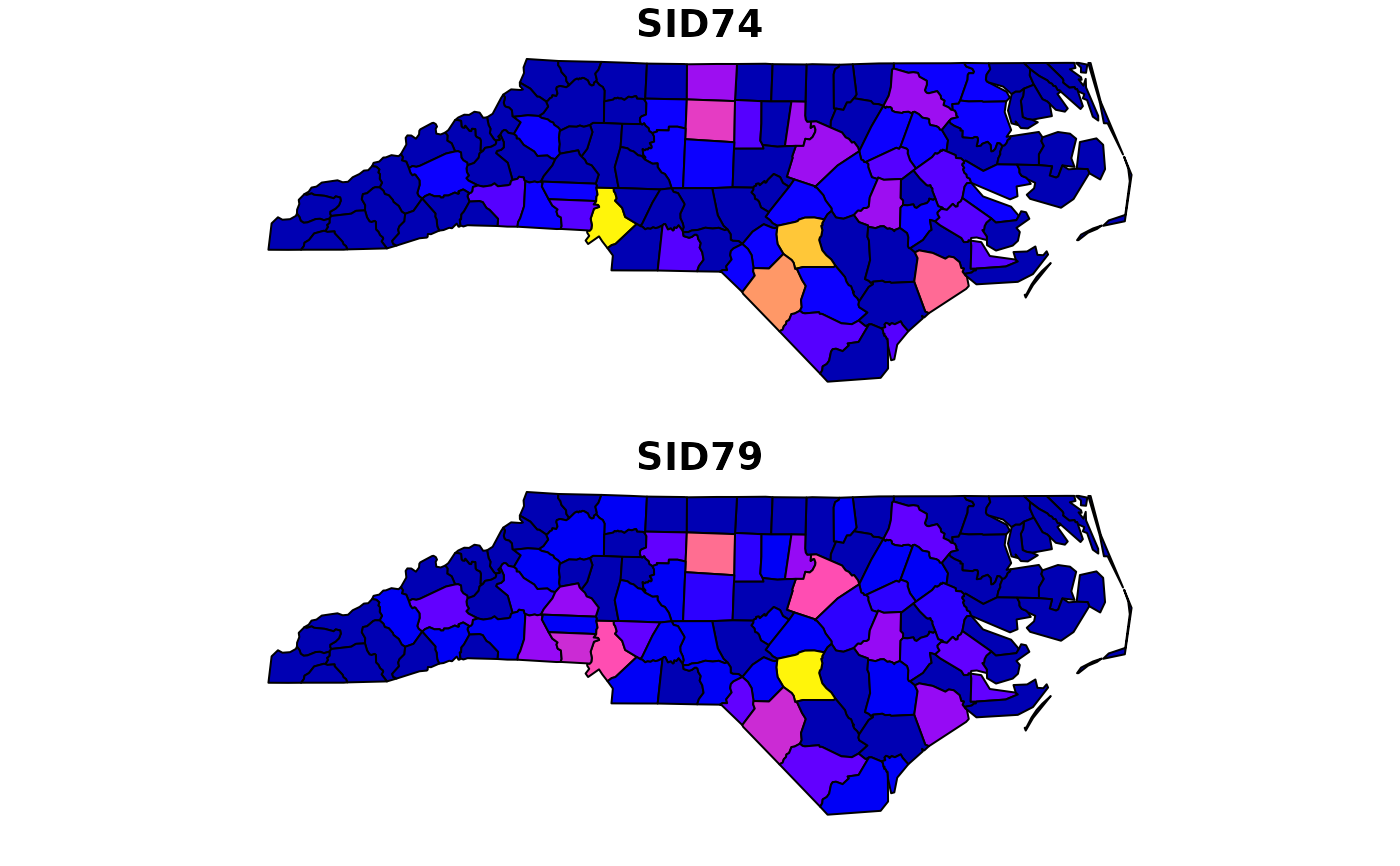# adding to a plot of an sf object only works when using reset=FALSE in the first plot:
plot(nc["SID74"], reset = FALSE)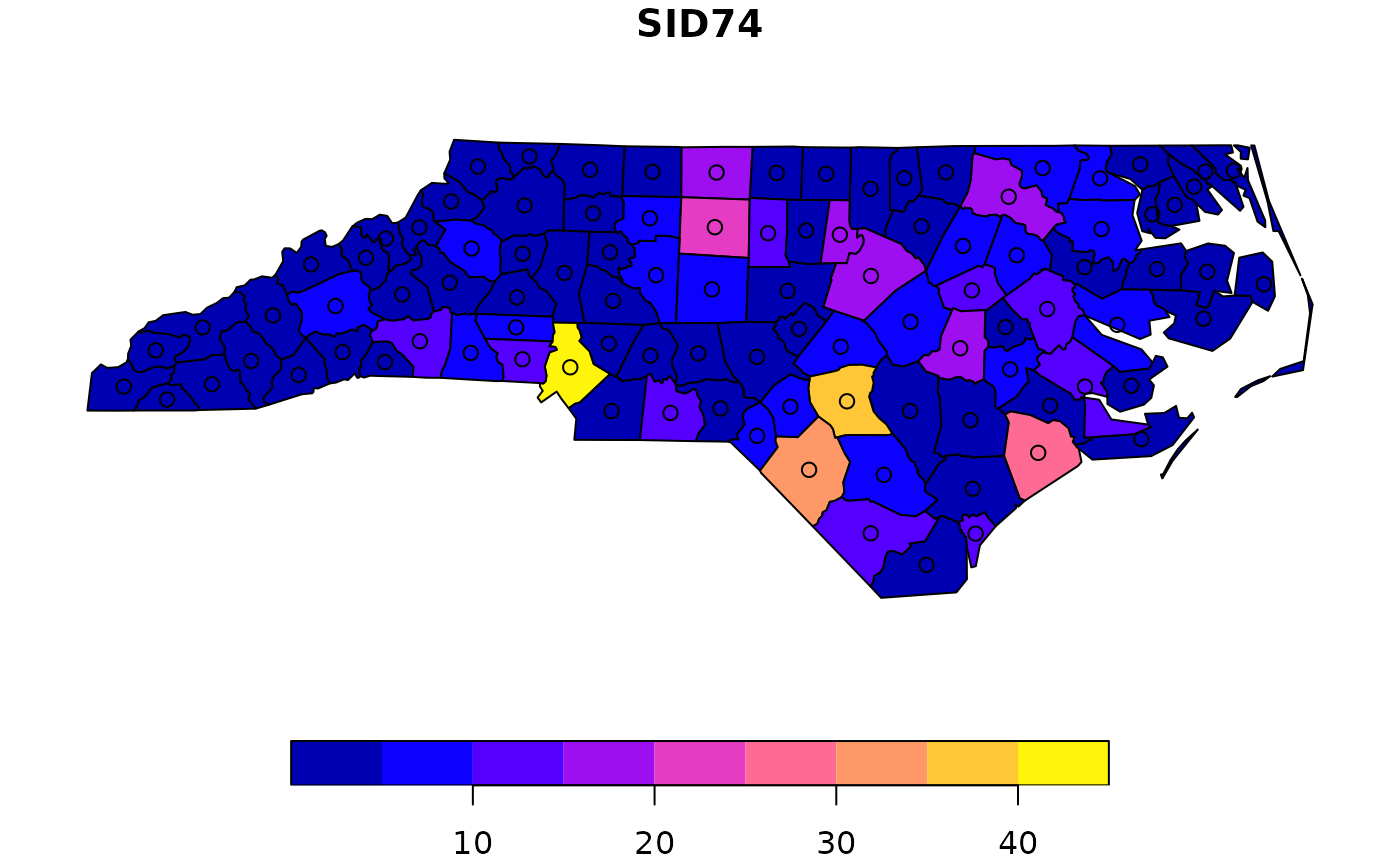# log10 z-scale:
plot(nc["SID74"], logz = TRUE, breaks = c(0,.5,1,1.5,2), at = c(0,.5,1,1.5,2))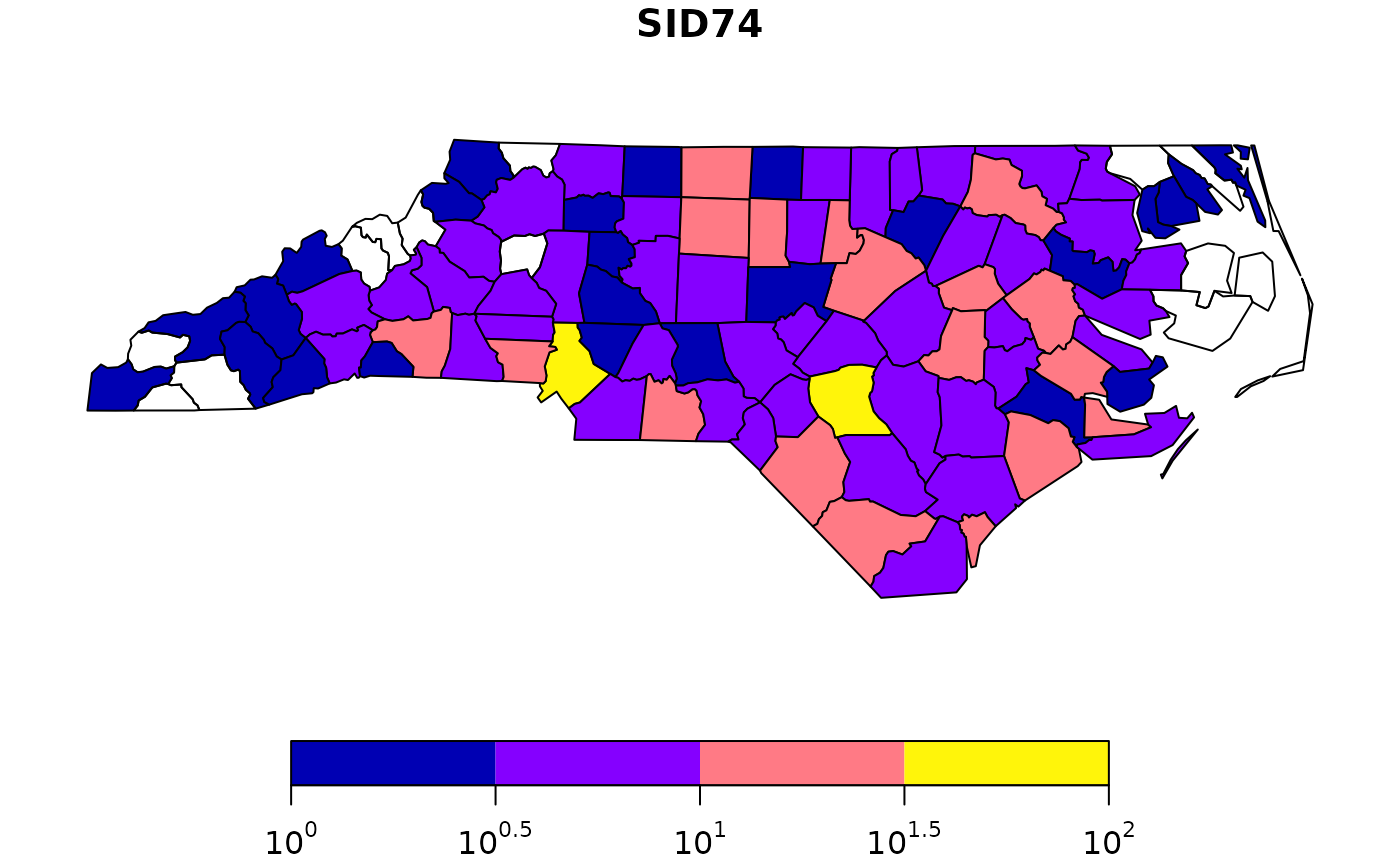# and we need to reset the plotting device after that, e.g. by
layout(1)
# when plotting only geometries, the reset=FALSE is not needed:
plot(st_geometry(nc))
plot(st_geometry(nc), col = 'red', add = TRUE)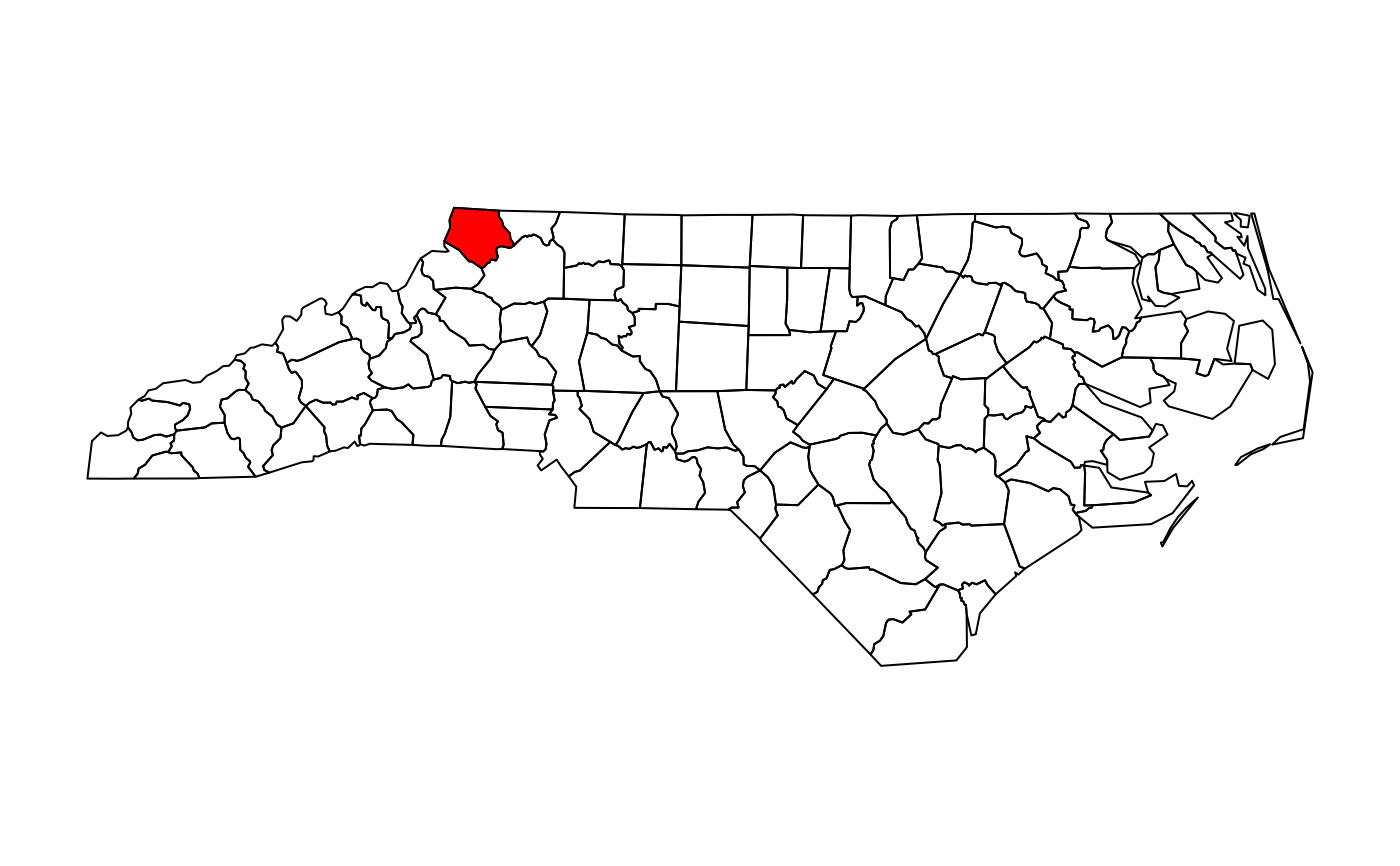# add a custom legend to an arbitray plot:
layout(matrix(1:2, ncol = 2), widths = c(1, lcm(2)))
plot(1)
.image_scale(1:10, col = sf.colors(9), key.length = lcm(8), key.pos = 4, at = 1:10)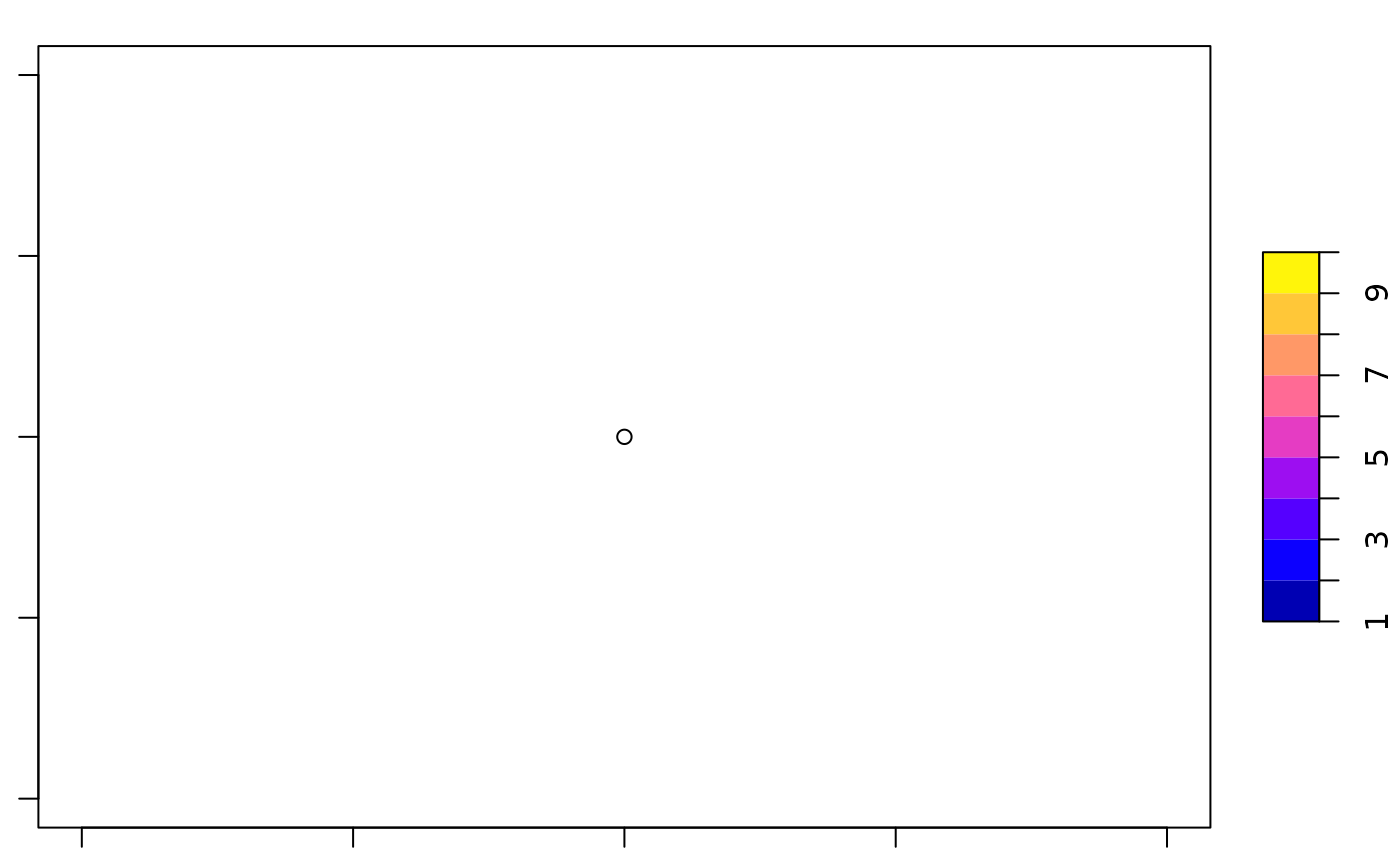# manipulate plotting order, plot largest polygons first:
p = st_polygon(list(rbind(c(0,0), c(1,0), c(1,1), c(0,1), c(0,0))))
x = st_sf(a=1:4, st_sfc(p, p * 2, p * 3, p * 4)) # plot(x, col=2:5) only shows the largest polygon!
plot(x[order(st_area(x), decreasing = TRUE),], col = 2:5) # plot largest polygons first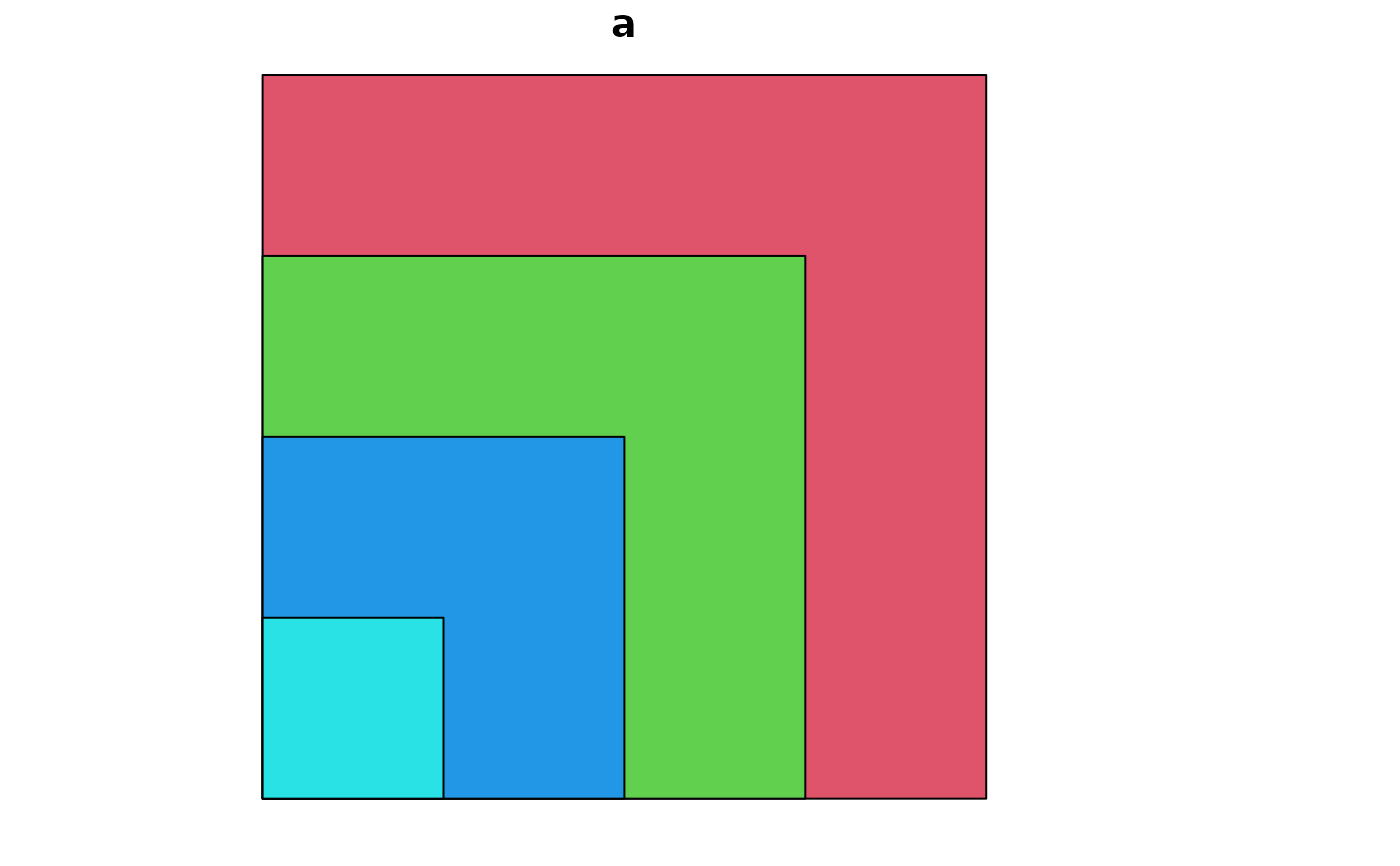sf.colors(10)
#>   "#0000B3FF" "#0400FFFF" "#4500FFFF" "#8500FFFF" "#C527D8FF" "#FF50AFFF"
#>   "#FF7A85FF" "#FFA35CFF" "#FFCC33FF" "#FFF50AFF"